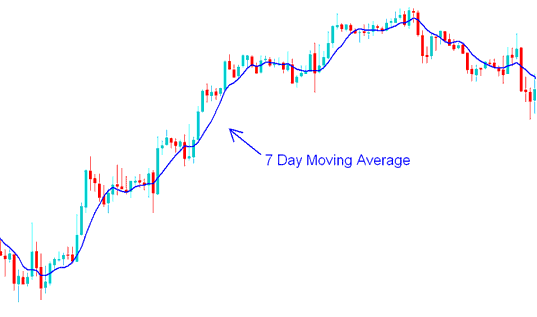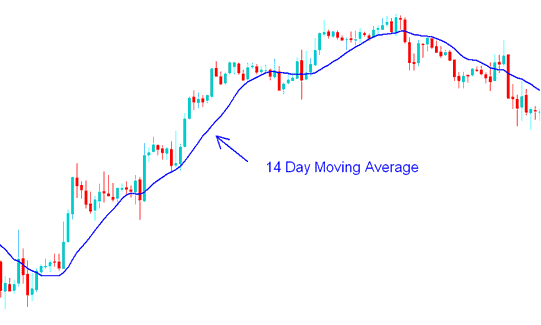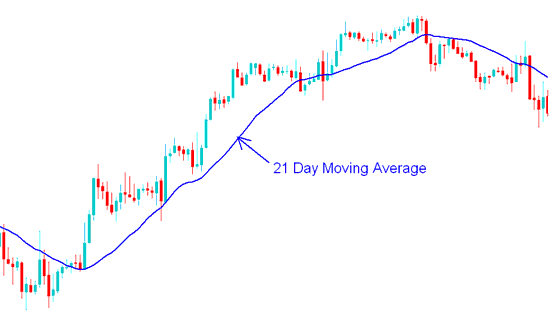# Trading Short-Term and Long-Term Indices Price Period of Moving Average

A stock indices trader can choose to adjust the stock index price periods used to calculate the moving average.

If a stock indices trader uses short stock index price periods then the MA will react faster to the changes in stock indices price.

For example if a stock indices trader uses the 7 day stock indices moving average then, the moving average indicator will react to the stock index price change much faster than a 14 day or 21 day stock indices Moving Average would. However, using short time stock index price periods to calculate the MA might result in the indicator giving false stock indices signals (whipsaws).7 Day Moving Average - Moving Average Stock Indices Strategies

If another trader uses longer time periods then the MA will react to stock index price changes much slower.

For example, if a stock indices trader uses the 14 day MA then the average will be less prone to whipsaws but it will react much slower.14 Day Moving Average - Moving Average Stock Indices Strategy Example21 Day Moving Average - Moving Average Stock Indices Strategies Example